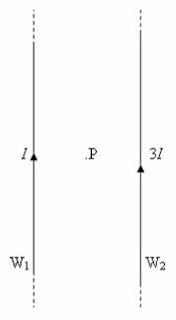## Pages

`“Life is like riding a bicycle.  To keep your balance you must keep moving.”–Albert Einstein`

## Monday, August 2, 2010

### Practice Questions (MCQ) on Magnetic Fields for AP Physics B & C

Questions involving magnetic fields posted on this site earlier can be accessed by clicking on the label ‘magnetic field’ below this post or by trying a search for ‘magnetic field’ using the search box provided on this page. Answering questions on magnetic field will not be generally difficult; but occasionally you will find questions consuming too much of your precious time. Today we will discuss a few more multiple choice questions involving magnetic fields:(1) Two straight long vertical wires W1 and W2 carry steady currents I and 3I respectively as shown. P is a point midway between the wires. The resultant magnetic flux density at P due to the currents in the wires is B. The direction of B is

(a) vertically upwards

(b) vertically downwards

(c) horizontal and directed normally into the plane of the figure (away from the reader)

(d) horizontal and directed normal to the plane of the figure, towards the reader

(e) horizontal and directed towards the wire W2

The magnetic field at P due to the current I in the wire W1 is directed normally into the plane of the figure (away from the reader). The magnetic field at P due to the current 3I in the wire W2 opposes the above field. Since the magnitude of the field due to the wire W2 is three times that due to the wire W1, the resultant field will be directed normal to the plane of the figure, towards the reader [Option (d)].

(2) In the above question if the current 3I in the wire W2 is switched off, what will be the magnetic field at P?

(a) B

(b) B

(c) B/2

(d) B/2

(e)B/3

The direction of the magnetic field produced by the current I in the wire W1 is opposite to the net field B in question No.(1). So the sign has to be negative. Since the currents I and 3I in the wires W1 and W2 produce opposing fields at P (in question no.1), the resultant field B has magnitude proportional to 2I. When the current 3I in the wire W2 is switched off, the field at P is proportional to I so that the magnitude is reduced to half the net value in question No.(1).

The field at P in this case is therefore B/2.

The following questions are specifically meant for AP Physics C aspirants:

(3) The magnetic flux density at the centre of a plane circular coil of radius R carrying a current I is B0. If the current in the coil is reversed, the magnetic flux density on the axis of the coil at distance R from the centre will be

(a) B0/√8

(b) B0/√2

(c) B0/√8

(d) B0/2

(e) B0/2

The magnitude of the magnetic flux density B at a point on the axis of the coil at distance x from the centre of the coil is given by

B = μ0nR2I/2(R2 + x2)3/2 where n is the number of turns in the coil.

The magnetic field B0 at the centre (x = 0) of the coil is given by

B0 = μ0nI/2R

The field (B1) on the axis at distance R from the centre (x = R) is given by

B1 = μ0nR2I/2(R2 + R2)3/2 = μ0nR2I/4√2 R3

Or, B1 = μ0nI/4√2 R

Therefore, B1 = B0/2√2 = B0/√8

Since the current in the coil is reversed, the direction of the magnetic field must be reversed so that the correct option is – B0/√8.

(4) An electron moving with velocity (30 i + 40 j) ms–1 enters a magnetic field of – 2 k tesla where i, j and k are unit vectors along the x, y and z directions respectively. Then

(a) the speed of the electron will not change; but the path will become parabolic

(b) the speed alone will change

(c) the speed will not change; but the path will become helical

(d) the speed will change and the path will become circular

(e) the speed will not change; but the path will become circular

Outside the magnetic field the path of the electron is straight and is contained in the xy plane. The magnetic field is directed along the negative z-direction an hence the electron enters normally into the magnetic field. A magnetic force acts perpendicular to the direction of motion of the electron.

The path of the electron within the magnetic field will therefore be circular. But the speed will be unchanged since a stationary magnetic field cannot change the kinetic energy of the electron. The correct option is (e).

[If the initial velocity of the electron had an z-component also (for instance, v = 30 i + 40 j + 20 k), the electron would enter the magnetic field at an angle other than 90º and then the path would be helical].